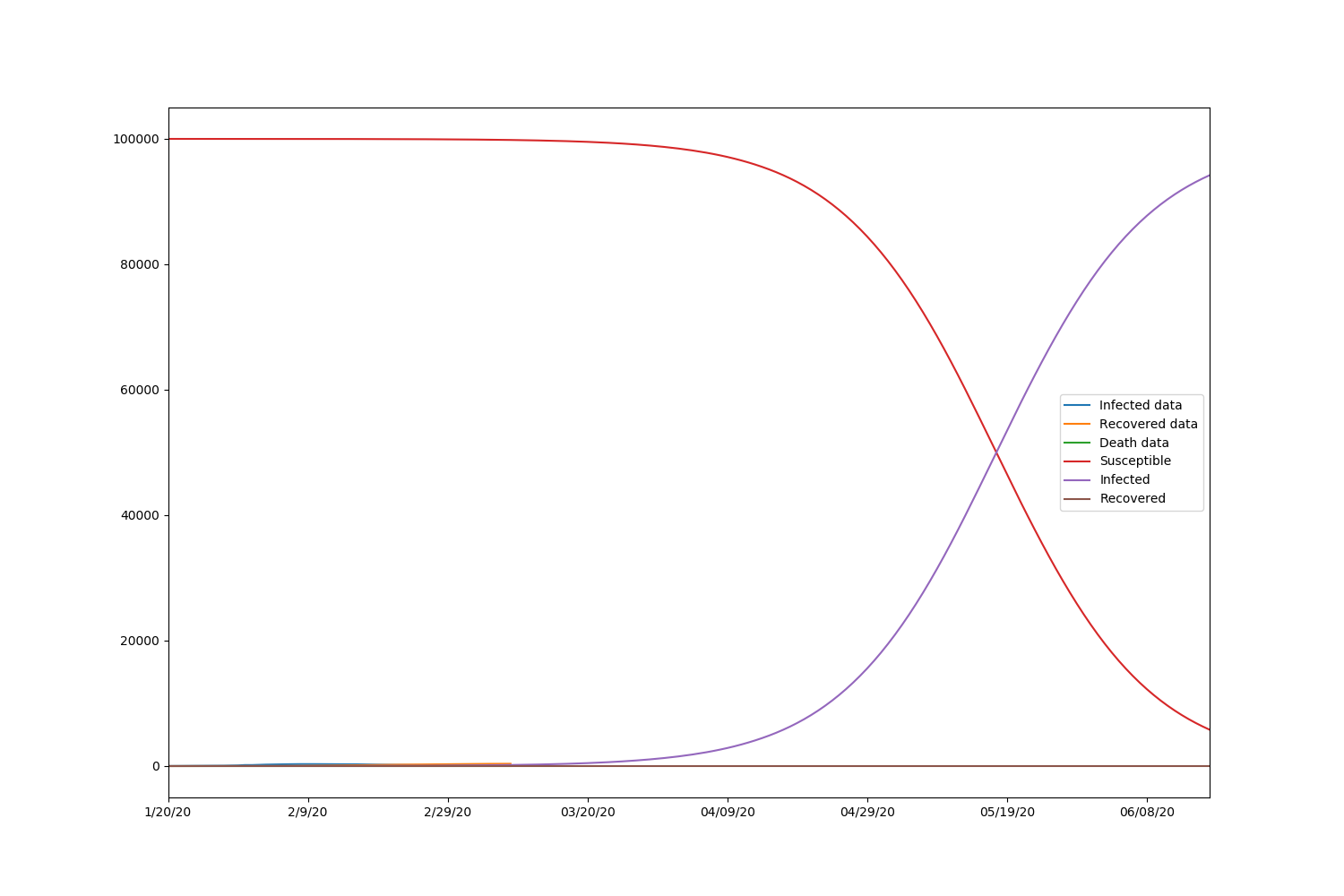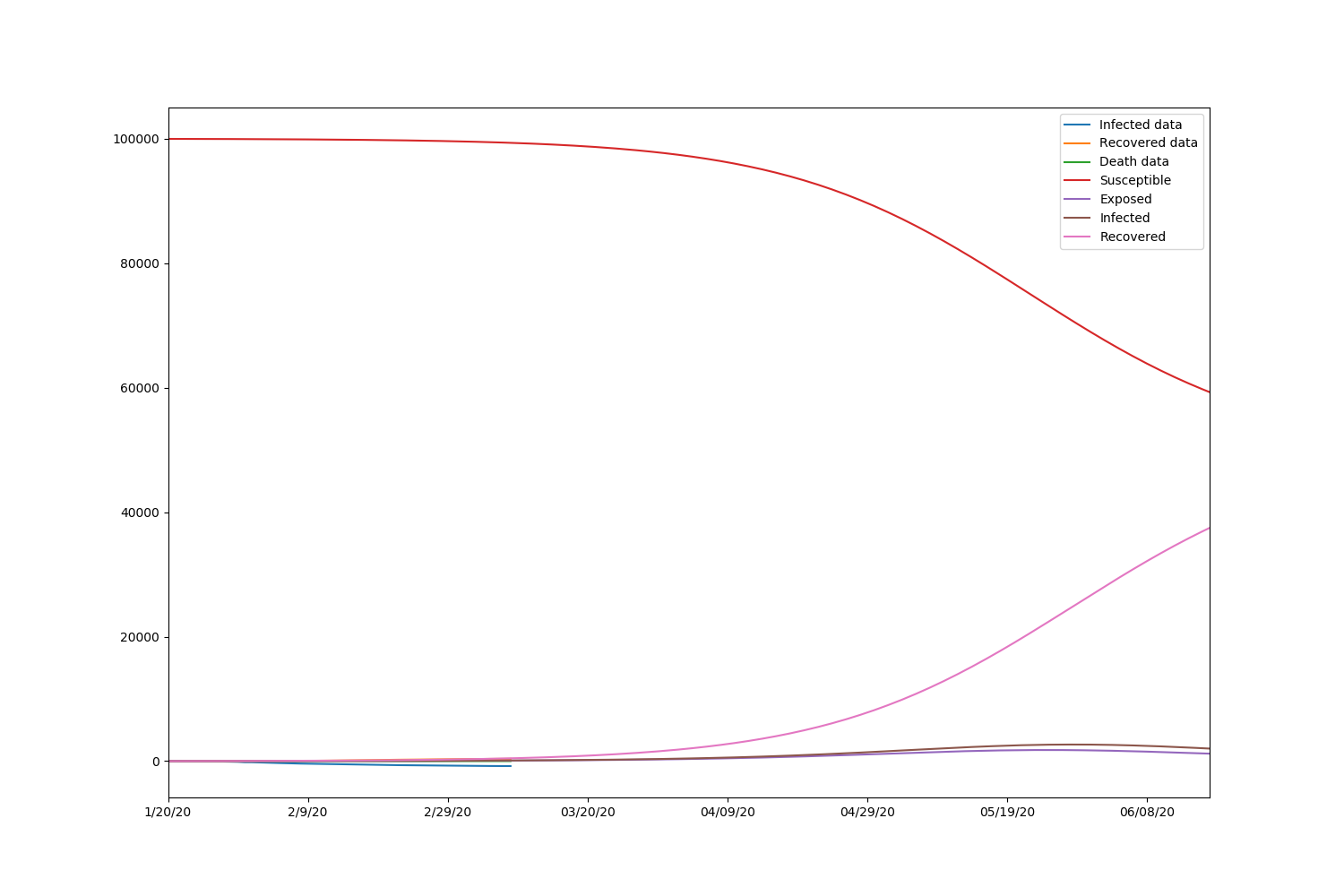github地址

# Deterministic Model for Disease Spreading

Typical deterministic model for disease spreading includes SIR mode and SEIR model.

## SIR model

The general SIR model takes s s as the ratio of susceptible people, i i as the infected people, r r as the recovered people. Then we have:
KaTeX parse error: No such environment: align at position 8: \begin{̲a̲l̲i̲g̲n̲}̲ &\frac{ds}{dt}…
Here, β \beta is the rate of transmission (transmissions per S-I contact per time), γ \gamma is the rate of recovery (inverse of infectious period). We could solve these equation and get:
s = s 0 e − β γ r s=s_0e^{-\frac{\beta}{\gamma}r}
With s + i + r = 1 s+i+r=1 , we could drive:
d r d t = γ ( 1 − r − s 0 e − β γ r ) t = 1 γ ∫ 0 r d u 1 − u − s 0 e − β γ u \frac{dr}{dt}=\gamma (1-r-s_0e^{-\frac{\beta}{\gamma}r})\\ t=\frac{1}{\gamma}\int_0^r\frac{du}{1-u-s_0e^{-\frac{\beta}{\gamma}u}}
With the above equations, we could fit the lines of the rate of susceptible, infectious and recovered people and get the parameter estimation of γ \gamma and β \beta .

Also, we could drive their iteration form with S = s N , I = i N , R = r N S=sN, I=iN, R=rN and let c c be the number of people one contact:
KaTeX parse error: No such environment: align at position 8: \begin{̲a̲l̲i̲g̲n̲}̲ & S_n=S_{n-1}-…

## SEIR model

The general SEIR model takes s s as the ratio of susceptible people, e e as the exposed people, i i as the infected people, r r as the recovered people. Then we have:
KaTeX parse error: No such environment: align at position 8: \begin{̲a̲l̲i̲g̲n̲}̲ &\frac{ds}{dt}…

Here, β \beta is the rate of transmission (transmissions per S-I contact per time), γ \gamma is the rate of recovery (inverse of infectious period), α \alpha is the rate of progression (inverse of incubation period). We could solve these equation and get:
s = s 0 e − β γ r s=s_0e^{-\frac{\beta}{\gamma}r}
With s + e + i + r = 1 s+e+i+r=1 , we could drive:
d r d t = γ ( 1 − e − r − s 0 e − β γ r ) t = 1 γ ∫ 0 r d u 1 − e − u − s 0 e − β γ u \frac{dr}{dt}=\gamma (1-e-r-s_0e^{-\frac{\beta}{\gamma}r})\\ t=\frac{1}{\gamma}\int_0^r\frac{du}{1-e-u-s_0e^{-\frac{\beta}{\gamma}u}}
With the above equations, we could fit the lines of the rate of susceptible, infectious and recovered people and get the parameter estimation of γ \gamma and β \beta .

Also, we could drive their iteration form with S = s N , E = e N , I = i N , R = r N S=sN, E=eN,I=iN, R=rN and let c c be the number of people one contact:

S n = S n − 1 − c β I n − 1 S n − 1 N E n = E n − 1 + c β I n − 1 S n − 1 N − α E n − 1 I n = I n − 1 + α E n − 1 − γ I n − 1 R n = R n − 1 + γ I n − 1 S_n=S_{n-1}-\frac{c\beta I_{n-1}S_{n-1}}{N}\\ E_n=E_{n-1}+c\beta I_{n-1}S_{n-1}N-\alpha E_{n-1}\\ I_n=I_{n-1}+\alpha E_{n-1}-\gamma I_{n-1}\\ R_n=R_{n-1}+\gamma I_{n-1}\\

# Experiments for Deterministic Model based on Real Data

## SIR model fitting

Here, we use the data from Jaunary 2end 2020 to May 9th 2020 of Shenzhen, including the cummulative confirmed cases, cummulative cured cases and cummulative dead cases. Here, we apply SIR model and fit the data of Shenzhen to get the s s the ratio of susceptible people, i i as the infected people, r r as the recovered people.

data=pd.read_excel("02_SZ_DailyCases.xlsx")
data.head()

CityDatecummulative confirmed casescummulative cured casescummulative dead cases
0Shenzhen2020-01-20900
1Shenzhen2020-01-211400
2Shenzhen2020-01-221500
3Shenzhen2020-01-231520
4Shenzhen2020-01-242020
Here, we fit the model by minimize the MSE of the solution of the derivative equations.

m i n i m i z e    α 1 ( ∑ i = 1 n ( s i ^ − s i ) 2 + ( 1 − α 1 ) ( ∑ i = 1 n ( r i ^ − r i ) S e t   α 1 = 0.1 minimize ~~\alpha_1 (\sum_{i=1}^n (\hat{s_i}-s_i)^2+(1-\alpha_1)(\sum_{i=1}^n (\hat{r_i}-r_i)\\ Set ~ \alpha_1=0.1

#!/usr/bin/python
import numpy as np
import pandas as pd
from scipy.integrate import solve_ivp
from scipy.optimize import minimize
import matplotlib.pyplot as plt
from datetime import timedelta, datetime

predict_range = 150
s_0=99998
i_0=2
r_0=0
class Learner(object):
def __init__(self, loss, predict_range, s_0, i_0, r_0):
self.loss = loss
self.predict_range = predict_range
self.s_0 = s_0
self.i_0 = i_0
self.r_0 = r_0
def load_confirmed(self):
df = pd.read_csv('./model_fit/02_SZ_DailyCases.csv')
df.set_index(["Date"], inplace=True)
dff=df["cummulative confirmed cases"]
return dff.T

def load_recovered(self):
df = pd.read_csv('./model_fit/02_SZ_DailyCases.csv')
df.set_index(["Date"], inplace=True)
dff = df["cummulative cured cases"]
return dff.T

def load_dead(self):
df = pd.read_csv('./model_fit/02_SZ_DailyCases.csv')
df.set_index(["Date"], inplace=True)
dff = df["cummulative dead cases"]
return dff.T

def extend_index(self, index, new_size):
values = index.values
current = datetime.strptime(index[-1], '%m/%d/%y')
while len(values) < new_size:
current = current + timedelta(days=1)
values = np.append(values, datetime.strftime(current, '%m/%d/%y'))
return values

def predict(self, beta, gamma, data, recovered, death, s_0, i_0, r_0):
new_index = self.extend_index(data.index, self.predict_range)
size = len(new_index)

def SIR(t, y):
S = y
I = y
R = y
return [-beta * S * I, beta * S * I - gamma * I, gamma * I]

extended_actual = np.concatenate((data.values, [None] * (size - len(data.values))))
extended_recovered = np.concatenate((recovered.values, [None] * (size - len(recovered.values))))
extended_death = np.concatenate((death.values, [None] * (size - len(death.values))))
return new_index, extended_actual, extended_recovered, extended_death, solve_ivp(SIR, [0, size],
[s_0, i_0, r_0],
t_eval=np.arange(0, size, 1))

def train(self):
recovered = self.load_recovered()
death = self.load_dead()
data = (self.load_confirmed() - recovered - death)

optimal = minimize(loss, [0.001, 0.001], args=(data, recovered, self.s_0, self.i_0, self.r_0),
method='L-BFGS-B', bounds=[(0.00000001, 0.4), (0.00000001, 0.4)])
print(optimal)
beta, gamma = optimal.x
new_index, extended_actual, extended_recovered, extended_death, prediction = self.predict(beta, gamma, data,
recovered, death,

self.s_0, self.i_0,
self.r_0)
df = pd.DataFrame(
{'Infected data': extended_actual, 'Recovered data': extended_recovered, 'Death data': extended_death,
'Susceptible': prediction.y, 'Infected': prediction.y, 'Recovered': prediction.y},
index=new_index)
fig, ax = plt.subplots(figsize=(15, 10))
#ax.set_title(self.country)
df.plot(ax=ax)
print(f" beta={beta:.8f}, gamma={gamma:.8f}, r_0:{(beta / gamma):.8f}")
fig.savefig("result.png")

def loss(point, data, recovered, s_0, i_0, r_0):
size = len(data)
beta, gamma = point

def SIR(t, y):
S = y
I = y
R = y
return [-beta * S * I, beta * S * I - gamma * I, gamma * I]

solution = solve_ivp(SIR, [0, size], [s_0, i_0, r_0], t_eval=np.arange(0, size, 1), vectorized=True)
l1 = np.sqrt(np.mean((solution.y - data) ** 2))
l2 = np.sqrt(np.mean((solution.y - recovered) ** 2))
alpha = 0.1
return alpha * l1 + (1 - alpha) * l2

def main():
learner = Learner(loss, predict_range, s_0, i_0, r_0)
learner.train()

if __name__ == '__main__':
main()

      fun: 192.0426614226907
hess_inv: <2x2 LbfgsInvHessProduct with dtype=float64>
jac: array([208799.102424  ,   -539.91772688])
message: b'CONVERGENCE: REL_REDUCTION_OF_F_<=_FACTR*EPSMCH'
nfev: 87
nit: 5
status: 0
success: True
x: array([9.12866787e-07, 2.39518331e-07])
beta=0.00000091, gamma=0.00000024, r_0:3.81126064


The results are beta=0.00000091, gamma=0.00000024, r_0:3.81126064

Also, we draw the graph with 150 days of prediction.## SEIR model fitting

For SEIR model, since we could not get the data of exposed people, we simpy assume that the exposed people is a static ratio of the confirmed cases. Here, we set it as 0.1 to get the model fit parameters.
m i n i m i z e      α 1 ( ∑ i = 1 n ( s i ^ − s i ) 2 + α 2 ( ∑ i = 1 n ( e i ^ − e i ) + ( 1 − α 1 − α 2 ) ( ∑ i = 1 n ( r i ^ − r i ) S e t   α 1 = 0.1 , α 2 = 0.1 minimize~~~~ \alpha_1 (\sum_{i=1}^n (\hat{s_i}-s_i)^2+\alpha_2(\sum_{i=1}^n (\hat{e_i}-e_i)+(1-\alpha_1-\alpha_2)(\sum_{i=1}^n (\hat{r_i}-r_i)\\ Set ~ \alpha_1=0.1,\alpha_2=0.1

#!/usr/bin/python
import numpy as np
import pandas as pd
from scipy.integrate import solve_ivp
from scipy.optimize import minimize
import matplotlib.pyplot as plt
from datetime import timedelta, datetime

predict_range = 150
s_0=99990
e_0=8
i_0=2
r_0=0
ratio=0.5
class Learner(object):
def __init__(self, loss, predict_range, s_0, e_0,i_0, r_0):
self.loss = loss
self.predict_range = predict_range
self.s_0 = s_0
self.e_0 = e_0
self.i_0 = i_0
self.r_0 = r_0

def load_confirmed(self):
df = pd.read_csv('./model_fit/02_SZ_DailyCases.csv')
df.set_index(["Date"], inplace=True)
dff=df["cummulative confirmed cases"]
return dff.T

def load_exposed(self,ratio):
df = pd.read_csv('./model_fit/02_SZ_DailyCases.csv')
df.set_index(["Date"], inplace=True)
dff=df["cummulative confirmed cases"]
dfff=dff*ratio
return dfff.T

def load_recovered(self):
df = pd.read_csv('./model_fit/02_SZ_DailyCases.csv')
df.set_index(["Date"], inplace=True)
dff = df["cummulative cured cases"]
return dff.T

def load_dead(self):
df = pd.read_csv('./model_fit/02_SZ_DailyCases.csv')
df.set_index(["Date"], inplace=True)
dff = df["cummulative dead cases"]
return dff.T

def extend_index(self, index, new_size):
values = index.values
current = datetime.strptime(index[-1], '%m/%d/%y')
while len(values) < new_size:
current = current + timedelta(days=1)
values = np.append(values, datetime.strftime(current, '%m/%d/%y'))
return values

def predict(self, beta, alpha, gamma, data, exposed, recovered, death, s_0, e_0, i_0, r_0):
new_index = self.extend_index(data.index, self.predict_range)
size = len(new_index)

def SEIR(t, y):
S = y
E = y
I = y
R = y
return [-beta * S * I, beta * S * I - alpha* E, alpha* E- gamma * I, gamma * I]

extended_actual = np.concatenate((data.values, [None] * (size - len(data.values))))
extended_exposed = np.concatenate((exposed.values, [None] * (size - len(exposed.values))))
extended_recovered = np.concatenate((recovered.values, [None] * (size - len(recovered.values))))
extended_death = np.concatenate((death.values, [None] * (size - len(death.values))))
return new_index, extended_actual, extended_exposed, extended_recovered, extended_death, solve_ivp(SEIR,[0, size],
[s_0, e_0,i_0, r_0],
t_eval=np.arange(0, size, 1))

def train(self):
recovered = self.load_recovered()
exposed = self.load_exposed(ratio)
death = self.load_dead()
data = (self.load_confirmed() - exposed - recovered - death)#易感人数

optimal = minimize(loss, [0.001, 0.001, 0.001], args=(data, exposed, recovered, self.s_0, self.e_0, self.i_0, self.r_0),
method='L-BFGS-B', bounds=[(0.00000001, 0.4), (0.00000001, 0.4), (0.00000001, 0.4)])
print(optimal)
beta, alpha, gamma = optimal.x
new_index, extended_actual, extended_exposed, extended_recovered, extended_death, prediction = self.predict(beta, alpha, gamma, data, exposed, recovered, death,

self.s_0, self.e_0,self.i_0,
self.r_0)
df = pd.DataFrame(
{'Infected data': extended_actual, 'Recovered data': extended_recovered, 'Death data': extended_death,
'Susceptible': prediction.y, 'Exposed': prediction.y, 'Infected': prediction.y, 'Recovered': prediction.y},
index=new_index)
fig, ax = plt.subplots(figsize=(15, 10))
#ax.set_title(self.country)
df.plot(ax=ax)
print(f" beta={beta:.8f}, alpha={alpha:.8f}, gamma={gamma:.8f}, r_0:{(beta / gamma):.8f}")
fig.savefig("result_SEIR.png")
fig.show()
print(df)

def loss(point, data, exposed, recovered, s_0, e_0, i_0, r_0):
size = len(data)
beta, alpha, gamma = point

def SEIR(t, y):
S = y
E = y
I = y
R = y
return [-beta * S * I, beta * S * I - alpha * E, alpha * E - gamma * I, gamma * I]

solution = solve_ivp(SEIR, [0, size], [s_0, e_0, i_0, r_0], t_eval=np.arange(0, size, 1), vectorized=True)
l1 = np.sqrt(np.mean((solution.y - data) ** 2))
l2 = np.sqrt(np.mean((solution.y - exposed) ** 2))
l3 = np.sqrt(np.mean((solution.y - recovered) ** 2))
a1 = 0.1
a2 = 0.1
return a1 * l1 + a2 * l2 + (1 - a1 - a2) * l3

def main():
learner = Learner(loss, predict_range, s_0, e_0, i_0, r_0)
learner.train()

if __name__ == '__main__':
main()

      fun: 111.62004441579865
hess_inv: <3x3 LbfgsInvHessProduct with dtype=float64>
jac: array([ 5.88108459e+06,  2.35953219e+02, -1.84868701e+02])
message: b'CONVERGENCE: REL_REDUCTION_OF_F_<=_FACTR*EPSMCH'
nfev: 180
nit: 16
status: 0
success: True
x: array([1.42325627e-05, 5.08089942e-02, 4.00000000e-01])
beta=0.00001423, alpha=0.05080899, gamma=0.40000000, r_0:0.00003558
Infected data Recovered data Death data   Susceptible      Exposed  \
1/20/20            4.5              0          0  99990.000000     8.000000
1/21/20              7              0          0  99987.374417    10.162146
1/22/20            7.5              0          0  99985.007648    11.965733
1/23/20            5.5              2          0  99982.704533    13.618636
1/24/20              8              2          0  99980.347766    15.242162
...                ...            ...        ...           ...          ...
06/13/20          None           None       None   5046.380713  9661.000189
06/14/20          None           None       None   4951.104209  9275.303313
06/15/20          None           None       None   4861.351492  8903.325929
06/16/20          None           None       None   4776.860612  8544.620430
06/17/20          None           None       None   4697.113617  8199.057671

Infected     Recovered
1/20/20      2.000000      0.000000
1/21/20      1.725445      0.737992
1/22/20      1.623365      1.403254
1/23/20      1.626192      2.050639
1/24/20      1.696952      2.713121
...               ...           ...
06/13/20  1363.575498  83929.043601
06/14/20  1308.856933  84464.735545
06/15/20  1256.480752  84978.841826
06/16/20  1206.930025  85471.588933
06/17/20  1159.089167  85944.739545

[150 rows x 7 columns]


The results are beta=0.00001423, alpha=0.05080899, gamma=0.40000000, r_0:0.00003558.

Also, we draw the graph with 150 days of prediction..02-116万+
09-092249
08-152383
07-303968
04-142708
08-11850
08-17
02-17
07-173347
05-072000
08-10
©️2020 CSDN 皮肤主题: 数字20 设计师:CSDN官方博客